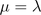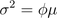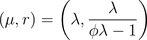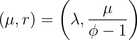Want to share your content on R-bloggers? click here if you have a blog, or here if you don't.

Here and there, I mentioned two codes to generated quasiPoisson random variables. And in both of them, the negative binomial approximation seems to be wrong. Recall that the negative binomial distribution iswhereand in R, a negative binomial distribution can be parametrized using two parameters, out of the following ones
• the size• the probability• the meanRecall also that the variance for a negative binomial distribution should beHere, we consider a distribution such thatand. In the previous posts, I usedi.e.
```rqpois = function(n, lambda, phi) {
mu = lambda
k = mu/(phi * mu - 1)
r1 = rnbinom(n, mu = mu, size = k)
r2 = rnbinom(n, size=phi*mu/(phi-1),prob=1/phi)
k = mu/phi/(1-1/phi)
r3 = rnbinom(n, mu = mu, size = k)
r4 = rnbinom(n, size=mu/phi/(1-1/phi),prob=1/phi)
r = cbind(r1,r2,r3,r4)
return(r)
}```
but as we can see below, none of those two functions work,
```> N=rqpois(1000000,2,4)
> mean(N[,1])
 2.001992
> mean(N[,2])
 8.000033
> var(N[,1])/ mean(N[,1])
 7.97444
> var(N[,2])/ mean(N[,2])
 4.002022```
with the first one, the expected value is correct, while it is the overdispersion parameter for the second. Now, if we do the maths correctly, it comeswhich should now work,
```> mean(N[,3])
 2.001667
> mean(N[,4])
 2.002776
> var(N[,3])/ mean(N[,3])
 3.999318
> var(N[,4])/ mean(N[,4])
 4.009647```
So, finally it is better when we do the maths well.